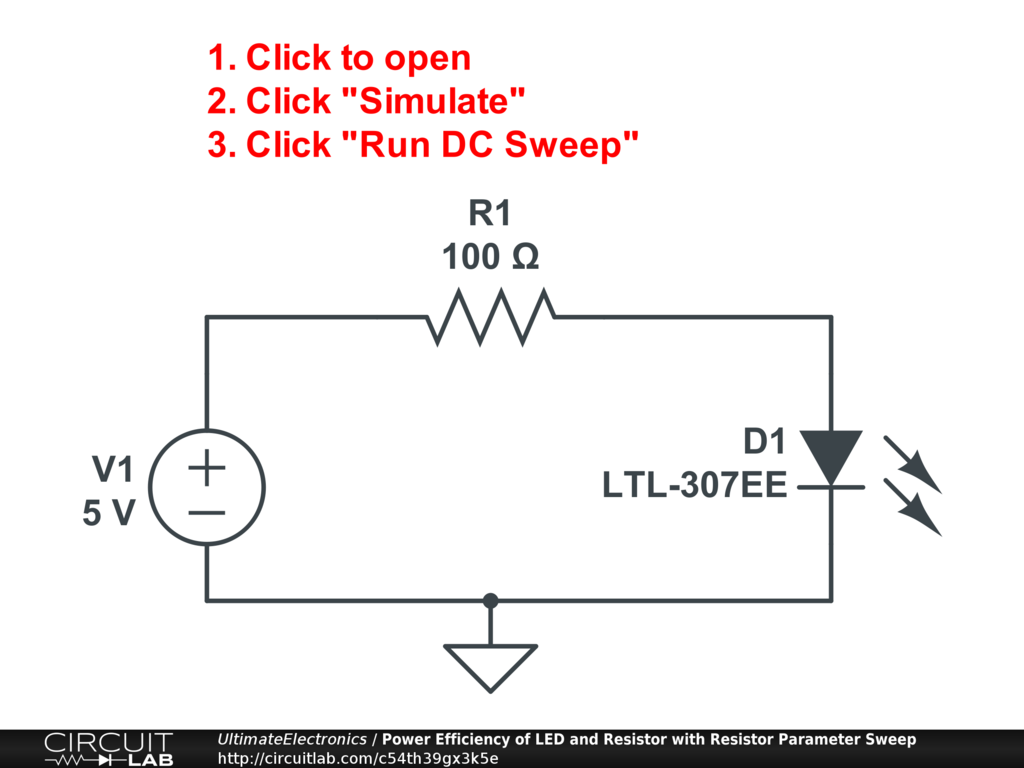Practical Resistors: Manufacturing Tolerances, Common Values, and Color Codes

Manufacturing Tolerances

Real resistors are not sold as exactly $100.00000 \ \Omega$ with 8 significant digits of precision. They're sold as, for example, $(100 \pm 5\%)\ \Omega$. Both a $95\ \Omega$ and a $105\ \Omega$ resistor would be acceptable under this specification.

Manufacturing tolerance has to do with manufacturing cost. If you want $\pm 0.1 \%$ precision resistors, you can get them, but they cost more. In some parts of your circuit design this will matter, and in others it won't. Simulation is a useful tool for rapidly making sure your circuit performs as expected for all possible extremes of each component.

Simulating Resistor Tolerance

It would be hard to test a wide range of component values (sometimes called "process corners") in the real world, but it's relatively easy in simulation.

Let's look at the LED plus resistor circuit we discussed briefly in the Power section, but we'll modify the simulation to repeat itself for values of the resistor from $95\ \Omega, \ 100\ \Omega, \ \text{and} \ 105\ \Omega$:Interactive Exercise Click the circuit, then click "Simulate," and "Run DC Sweep." We've used the second sweep parameter to adjust the parameter "R1.R", which causes the simulator to repeat the simulation for each specified resistance.

This provides a quick way, for example, to verify that the current doesn't exceed a certain limit, or that the efficiency is still reasonable, over the expected component variation. And this task takes a small fraction of a second in simulation.

Common Values

Some resistor values are common. Others are not. You can buy a $100\ \Omega$ resistor, but you can't easily buy a $104.357\ \Omega$ resistor.

If you truly needed a $104.357\ \Omega$ resistor in your circuit, you would probably have to make one by combining multiple resistors in series and parallel and/or using an adjustable resistor called a potentiometer.

Just as in any commodity part market, common size standards have emerged for compatibility and cost savings.

Some common 5% tolerance resistor values are: 10, 11, 12, 13, 15, 16, 18, 20, 22, 24, 27, 30, 33, 36, 39, 43, 47, 51, 56, 62, 68, 75, 82, 91. These are then multiplied by a power-of-ten scale, generally ranging from $10^{-1} \ \text{to} \ 10^5$. So you could easily purchase a $18 \times 10^{-1} = 1.8\ \Omega$ resistor, or a $47 \times 10^5 = 4.7\ \text{M} \Omega$ resistor.

Something interesting is that you'll find that if you look $\pm 5\%$ from any of these values, you'll find that you are within the $\pm 5\%$ band of the next nearest value! This means that all possible resistors can be assigned to one bin or another. This eliminates any manufacturing waste from a "bad batch," as the parts can be measured, sorted, and labeled after manufacturing.

Color Bands and Resistor Marking

We haven't yet covered Electronics Packaging, but most resistors are sold either as axial through-hole components (with a wire lead sticking out of either end) or as a surface mount component, like a tiny rectangle the size of a grain of rice.

Axial through-hole resistors typically have color bands. You can look these up to decode the color code. It may be useful to memorize the color code, but there is never any harm in simply double-checking with a multimeter before you use a resistor.

Surface mount resistors have printed codes with a sequence of numbers and letters. Beware, though: there are at least two different sequences used for coding surface mount resistors. In one system, the code might be "753" to indicate a $75 \times 10^3 \ \Omega$ resistor. In the other, the exact same resistance might be labeled "85C". To make things worse, it's easy to confuse a surface mount capacitor with a surface mount resistor. When in doubt, just use a multimeter to measure!

Matching

Sometimes, it's more important that two resistors are matched closely to each other than that their absolute resistance is any particular number.

When necessary, matching can be achieved with even more precision than finding a single precision resistor alone. When you think you want precision, think carefully: sometimes you really just want matching.

You can do decent matching with a bag of resistors by hand: pick 10-20 resistors, measure all of them, and then pick the closest two. It's not hard to take a bag of same-valued $\pm 5\%$ tolerance resistors and end up with a matched pair that's $\pm 0.2\%$ or so with just an ordinary multimeter.

You can do very good matching by adding a potentiometer in series with one of the resistors and adjusting it until the two legs match in resistance.

You can do even better matching with a bridge circuit, but we'll leave that for later.

What's Next

In the next section, Practical Resistors: Power Rating (Wattage), we'll talk about the fact that resistors turn electrical energy into heat, and that heat has to go somewhere before the resistors melts!